Products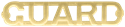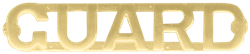### A1013

(image does not represent actual size)
Dimensions: 1 1/2" (W) x 5/16" (H)
A1013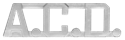### A10262

(image does not represent actual size)
Dimensions: 1 1/8" (W) x 5/16" (H)
A10262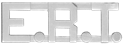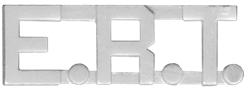### A10315

(image does not represent actual size)
Dimensions: 1 1/8" (W) x 3/8" (H)
A10315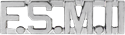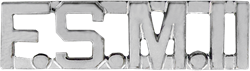### A10316

(image does not represent actual size)
Dimensions: 1 3/8" (W) x 3/8" (H)
A10316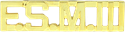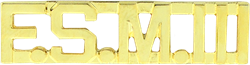### A10317

(image does not represent actual size)
Dimensions: 1 1/2" (W) x 3/8" (H)
A10317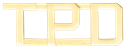### A10376

(image does not represent actual size)
Dimensions: 1 3/8" (W) x 1/2" (H)
A10376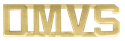### A10394

(image does not represent actual size)
Dimensions: 1 1/8" (W) x 5/16" (H)
A10394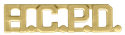### A10428

(image does not represent actual size)
Dimensions: 1 3/8" (W) x 5/16" (H)
A10428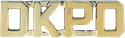### A10544

(image does not represent actual size)
Dimensions: 1" (W) x 5/16" (H)
A10544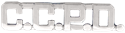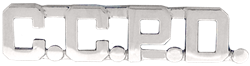### A10756

(image does not represent actual size)
Dimensions: 1 3/8" (W) x 5/16" (H)
A10756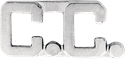### A10782

(image does not represent actual size)
Dimensions: 3/4" (W) x 3/8" (H)
A10782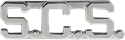### A10819

(image does not represent actual size)
Dimensions: 1 5/8" (W) x 1/2" (H)
A10819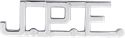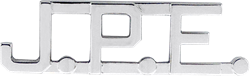### A10882

(image does not represent actual size)
Dimensions: 1 3/4" (W) x 1/2" (H)
A10882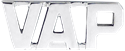### A11031

(image does not represent actual size)
Dimensions: 1" (W) x 3/8" (H)
A11031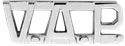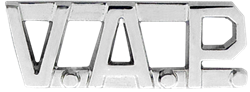### A11032

(image does not represent actual size)
Dimensions: 1" (W) x 3/8" (H)
A11032